Question

# Using VSEPR theory, a molecule with the general formula AX E# (A is the central atom,...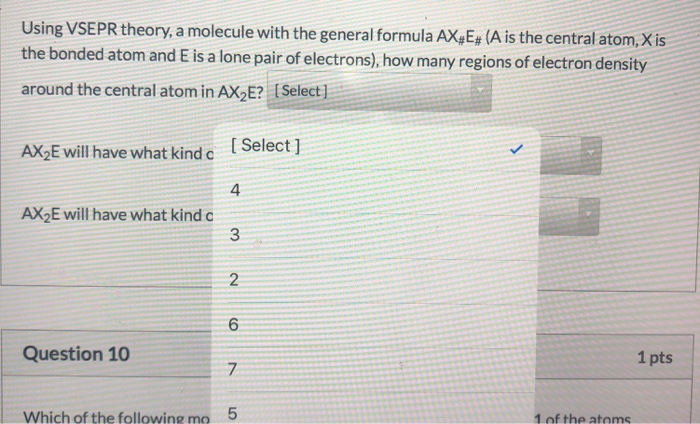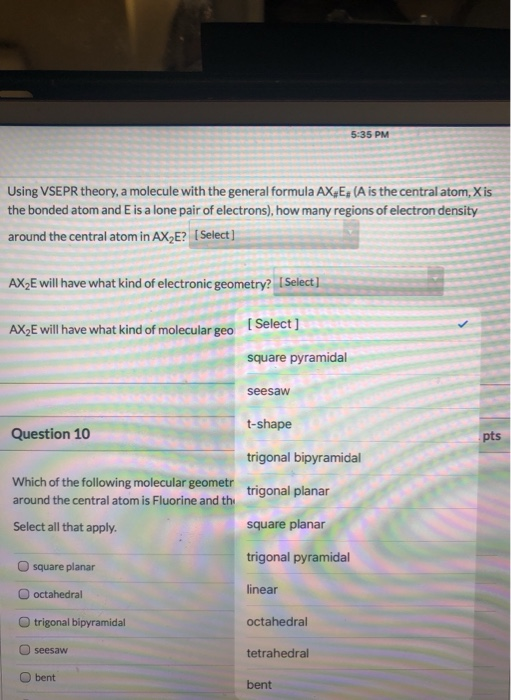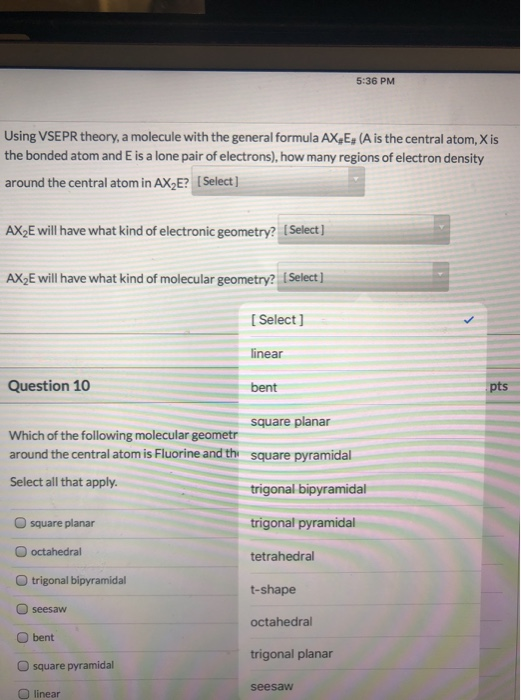Using VSEPR theory, a molecule with the general formula AX E# (A is the central atom, Xis the bonded atom and E is a lone pair of electrons), how many regions of electron density around the central atom in AX E? [Select] AX2E will have what kind c [Select] AX2E will have what kind a w N o Question 10 1 pts V Which of the following mo 5 1 of the atoms
5:35 PM Using VSEPR theory, a molecule with the general formula AX E, (A is the central atom, X is the bonded atom and E is a lone pair of electrons), how many regions of electron density around the central atom in AX E? [Select] AX2E will have what kind of electronic geometry? [Select] AXzE will have what kind of molecular Reo [ Select] square pyramidal seesaw t-shape Question 10 trigonal bipyramidal Which of the following molecular geometr around the central atom is Fluorine and thi trigonal planar Select all that apply. square planar trigonal pyramidal square planar octahedral linear octahedral trigonal bipyramidal seesaw tetrahedral O bent bent
5:36 PM Using VSEPR theory, a molecule with the general formula AX,E, (A is the central atom, Xis the bonded atom and E is a lone pair of electrons), how many regions of electron density around the central atom in AX2E? [Select] AX2E will have what kind of electronic geometry? (Select) AX2E will have what kind of molecular geometry? (Select] [ Select ] linear Question 10 bent square planar Which of the following molecular geometr around the central atom is Fluorine and the square pyramidal Select all that apply. trigonal bipyramidal square planar trigonal pyramidal octahedral tetrahedral trigonal bipyramidal t-shape seesaw octahedral bent trigonal planar square pyramidal seesaw linear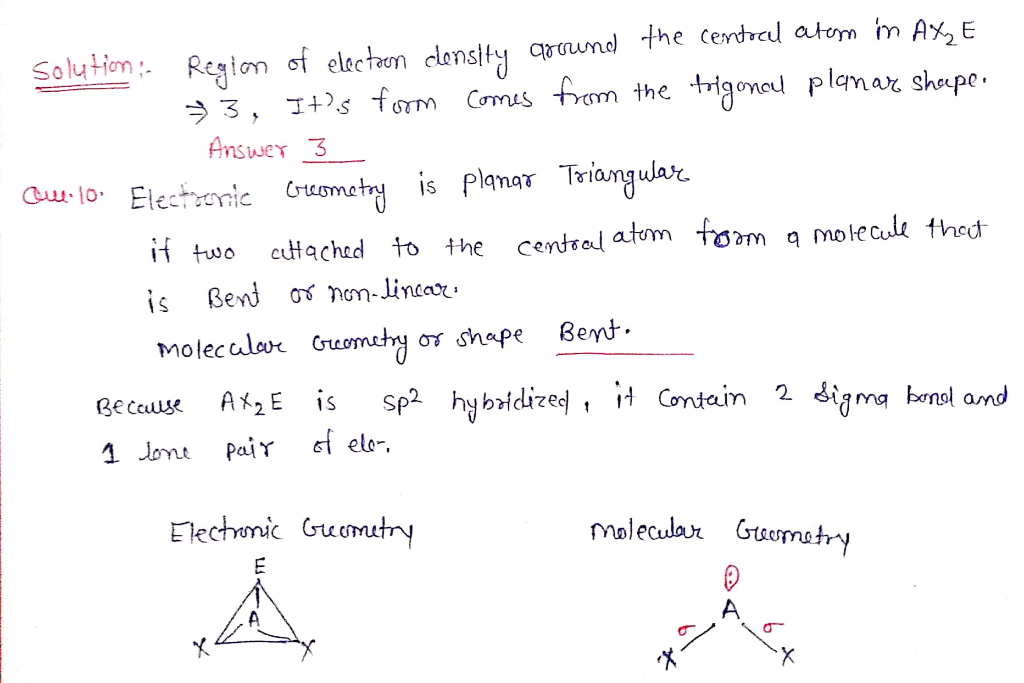#### Earn Coins

Coins can be redeemed for fabulous gifts.

Similar Homework Help Questions
• ### According to VSEPR theory, XeO3 has how many regions of electron density around the central atom...

According to VSEPR theory, XeO3 has how many regions of electron density around the central atom (how many electron groups?) (enter a number from 1 to 6) ? XeO3 will have (linear, trigonal planar, tetrahedral, trigonal pyramidal, bent, trigonal bipyramidal, seesaw, T-shaped, octahedral, square pyramidal, square planar) electronic geometry, and (linear, trigonal planar, tetrahedral, trigonal pyramidal, bent, trigonal bipyramidal, seesaw, T-shaped, octahedral, square pyramidal, square planar) molecular geometry. Draw the best Lewis structure using formal charge information. How many single...

• ### According to VSEPR theory, a molecule with the general formula AX2E (A is the central atom,...

According to VSEPR theory, a molecule with the general formula AX2E (A is the central atom, X is the bonded atom and E is a lone pair of electrons) has how many regions of electron density around the central atom ? AX2E will have what kind of electronic geometry? AX2E will have what kind of molecular geometry?

• ### VSEPR theory predicts which shape for the molecular structure of the following molecules or molecular ions?...

VSEPR theory predicts which shape for the molecular structure of the following molecules or molecular ions? (i.e. the arrangement of only the bonded atoms about the central atom) bent (or angular) linear octahedral see-saw square planar square pyramidal tetrahedral trigonal bipyramidal trigonal planar trigonal pyramidal T-shaped  ICl3 molecule    (The central atom is I.) bent (or angular) linear octahedral see-saw square planar square pyramidal tetrahedral trigonal bipyramidal trigonal planar trigonal pyramidal T-shaped  IO4- ion    (The central atom is I.) bent (or angular) linear...

• ### Provide the correct molecular geometry for (c), given the number of lone pairs and bonding groups...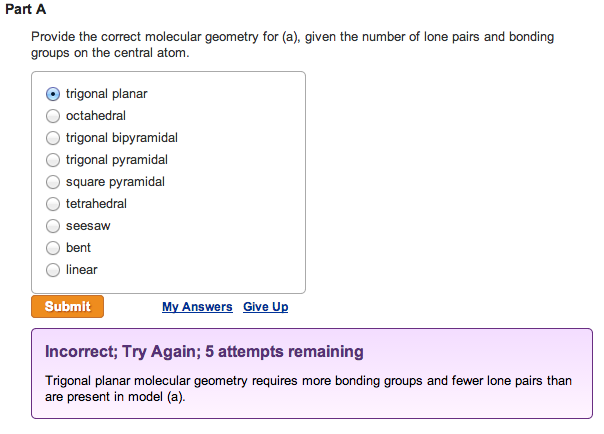Provide the correct molecular geometry for (a), given the number of lone pairs and bonding groups on the central atom. trigonal planar  octahedral  trigonal bipyramidal  trigonal pyramidal  square pyramidal  tetrahedral  seesaw  bent  linearProvide the correct molecular geometry for (b), given the number of lone pairs and bonding groups on the central atom.  trigonal bipyramidal  square pyramidal  bent  tetrahedral  trigonal planar  seesaw  trigonal pyramidal  octahedral  linear Provide the correct molecular geometry for (c), given the number of lone pairs and bonding groups on the...

• ### Number 1-6 1. What is the molecular geometry of SBr4? A) seesaw B) square planar C)...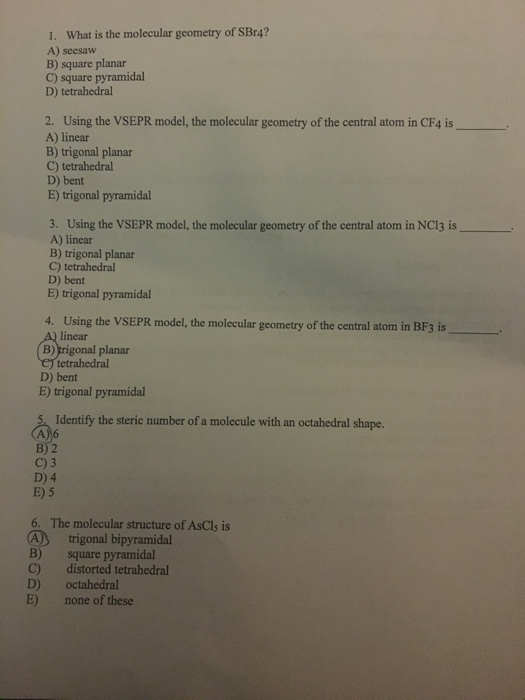Number 1-6 1. What is the molecular geometry of SBr4? A) seesaw B) square planar C) square pyramidal D) tetrahedral 2. Using the VSEPR model, the molecular geometry of the central atom in CF4 is A) linear B) trigonal planar C) tetrahedral D) bent E) trigonal pyramidal 3. Using the VSEPR model, the molecular geometry of the central atom in NC13 is A) linear B) trigonal planar C) tetrahedral D) bent E) trigonal pyramidal 4. Using the VSEPR model, the...

• ### 10.) Specify the electron-domain geometry for the following two species using the VSEPR model: Xe...

10.) Specify the electron-domain geometry for the following two species using the VSEPR model: XeCl4 and IO4−. For each species, predict the bond angles based on the electron-domain geometry. (a)    XeCl4 geometry: linear trigonal planar    bent tetrahedral trigonal pyramidal trigonal bipyramidal seesaw T-shaped octahedral square pyramidal square planar pentagonal bipyramidal pentagonal pyramidal pentagonal planar bond angle: 60° 72°   90° 109.5° 120° 180° (b)    IO4− geometry: linear trigonal planar    bent tetrahedral trigonal pyramidal trigonal bipyramidal seesaw T-shaped octahedral square pyramidal square...

• ### Specify the electron-domain geometry for the following two species using the VSEPR model: KrCl4 and BrO4....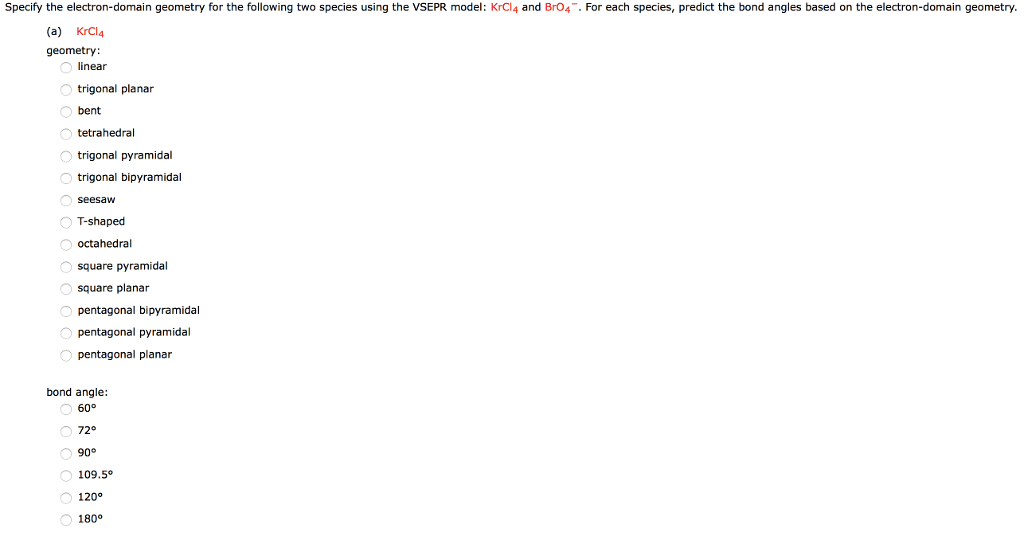Specify the electron-domain geometry for the following two species using the VSEPR model: KrCl4 and BrO4. For each species, predict the bond angles based on the electron-domain geometry. (a) KrCl4 geometry linear trigonal planar bent tetrahedral trigonal pyramidal trigonal bipyramidal T-shaped octahedral square pyramidal square planar pentagonal bipyramidal pentagonal pyramidal pentagonal planar bond angle: 72° 109.5° 120 180° (b) Br04 geometry: inear trigonal planar bent tetrahedral trigonal pyramidal trigonal bipyramidal seesaW T-shaped octahedral square pyramidal square planar pentagonal bipyramidal pentagonal...

• ### Which of the following molecular geometries (shapes) are always Polar if 1 of the atoms around the central atom is...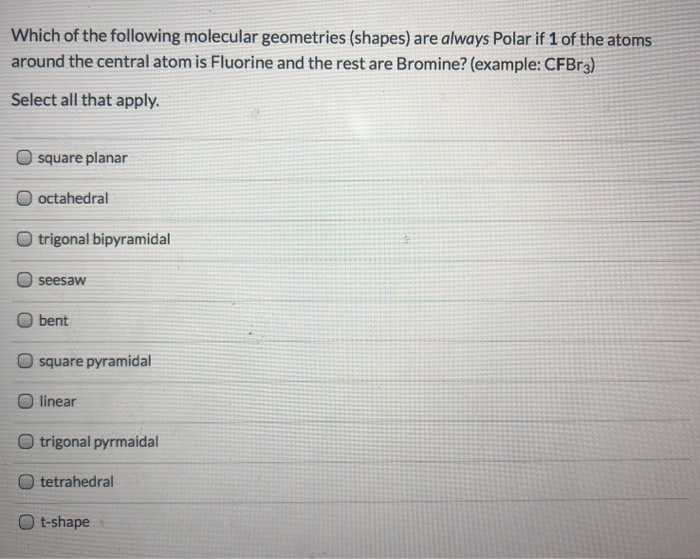Which of the following molecular geometries (shapes) are always Polar if 1 of the atoms around the central atom is Fluorine and the rest are Bromine? (example: CFBr3) Select all that apply. square planar O octahedral trigonal bipyramidal seesaw bent square pyramidal O linear trigonal pyrmaidal tetrahedral t-shape

• ### Part A Using the VSEPR model, the molecular geometry of the central atom in BF2 is...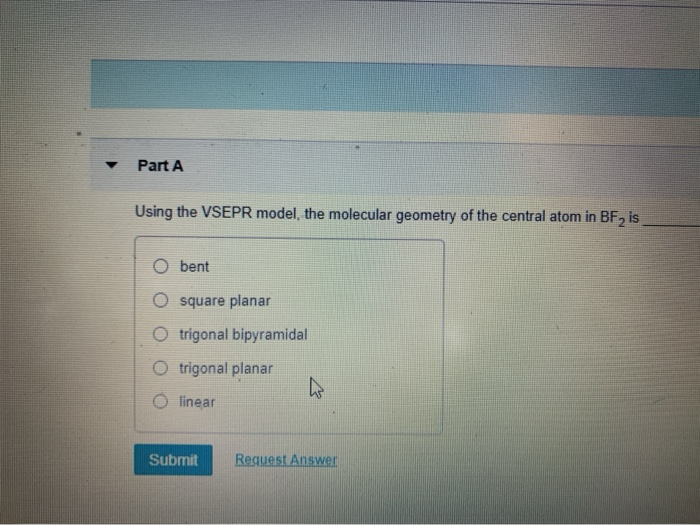Part A Using the VSEPR model, the molecular geometry of the central atom in BF2 is bent 0 square planar O trigonal bipyramidal trigonal planar h O linear Submit Request Answer

• ### olecular Geometry Saved Help Save & E Ch. Ex. 6 - Predict the Geometry Using VSEPR...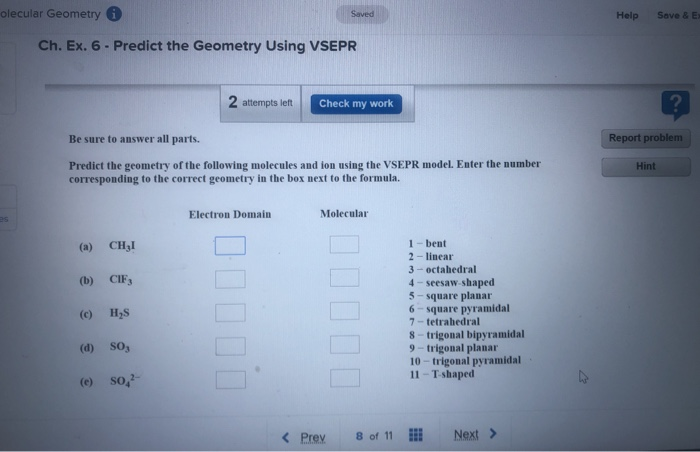olecular Geometry Saved Help Save & E Ch. Ex. 6 - Predict the Geometry Using VSEPR 2 attempts left Check my work Be sure to answer all parts. Report problem Hint Predict the geometry of the following molecules and ion using the VSEPR model. Enter the number corresponding to the correct geometry in the box next to the formula Electron Domain Molecular CHI 1 - bent 2 - linear 3-octahedral 4 - seesaw-shaped 5-square planar 6 - square pyramidal 7-tetrahedral...